# Generalized solution

(diff) ← Older revision | Latest revision (diff) | Newer revision → (diff)

A generalization of the concept of a classical solution of a differential (pseudo-differential) equation. It arose in relation to many problems in mathematical physics where it was necessary to regard as solutions of differential equations insufficiently differentiable functions, or even nowhere-differentiable functions, or even more general objects such as generalized functions, hyperfunctions, etc. Thus, the concept of a generalized solution is closely related to those of a generalized derivative and a generalized function. The concept of a generalized solution goes back to L. Euler .

A generalized solution of the differential equation(1)in the classis any generalized functionin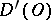satisfying equation (1) in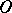, that is, for any test function, the equation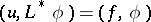must be satisfied. Hereis the operator adjoint toin the sense of Lagrange: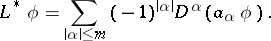A generalized solution of a boundary value problem should satisfy the boundary condition in the appropriate generalized sense (inor, etc.), for example: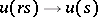,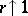, in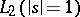;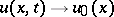,, in.

Generalized solutions of boundary value problems for differential equations arise when the latter are solved by variational methods, when applying difference methods, and also as weak limits of classical solutions when applying the Fourier method, the limiting-amplitude principle, pseudo-viscosity methods, etc.

### Examples.

1) The general solution of the equation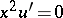in the class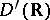is given by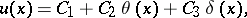whereis the Heaviside function: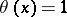,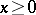;,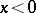;is the Dirac delta-function, andhere and below are arbitrary constants.

2) The equation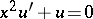has a single solution, equal to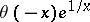, in the class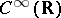, but in the class of hyperfunctions its general solution is given by.

3) The general solution of the wave equationin the classis given by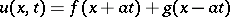, whereandare arbitrary functions of class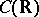.

4) Every solutioninof the Laplace equation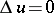is (real) analytic in.

5) Every solutioninof the heat equation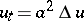is infinitely differentiable.

6) Every differential operator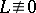with constant coefficients has a fundamental solution of slow growth (in the class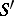).

7) Every equation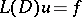, where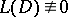is a differential operator with constant coefficients, has a generalized solutionin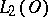for anyin, ifis a bounded domain.

8) A generalized solutionof the boundary value problem(2)

in the Sobolev class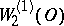arises as a solution of the classical variational problem of the minimum of the quadratic functionalin the class. The solution of this variational problem exists and is unique infor anyin. Thus, the generalized solution of (2) gives, for all, a self-adjoint extension of the operator(a so-called rigid or Friedrichs extension). The generalized solution of (2) together with its first derivatives are regular in(that is, are of the type of locally integrable functions on); its second derivatives are, generally speaking, singular generalized functions.

How to Cite This Entry:
Generalized solution. Encyclopedia of Mathematics. URL: http://encyclopediaofmath.org/index.php?title=Generalized_solution&oldid=17540
This article was adapted from an original article by V.S. Vladimirov (originator), which appeared in Encyclopedia of Mathematics - ISBN 1402006098. See original article